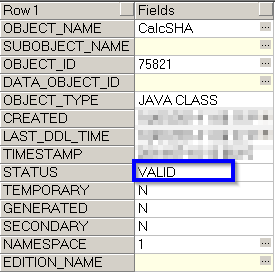# Create SHA-256 Hash - Oracle PL SQL Example

This post explains how you can create SHA-256 hash for a column / string in Oracle using PL SQL and Java Stored procedures with an example. You would need to have a basic understanding of creating Java stored procedures in Oracle to understand this tutorial. Also recommended is our tutorial to create SHA-256 for a file in Java (though not mandatory). Here is a step by step guide that explains how to get this working in Oracle Database;

## 1. Create Java Class to compute SHA-256 Hash for a String

In this step, we will write a simple Java class that has a method to calculate SHA-256 hash for a column in Oracle. You have to compile the class using the same Java version that is used by your database. The source code is provided below:
```import java.security.MessageDigest;
public class CalcSHA
{
public static String SHAVal(String inputVal) throws Exception
{
MessageDigest myDigest = MessageDigest.getInstance("SHA-256");
myDigest.update(inputVal.getBytes());
byte[] dataBytes = myDigest.digest();
StringBuffer sb = new StringBuffer();
for (int i = 0; i < dataBytes.length; i++) {
sb.append(Integer.toString((dataBytes[i] & 0xff) + 0x100, 16).substring(1));
}
return sb.toString();
}
}
```

The method SHAVal accepts a string (which we will pass from our SQL later) and returns the computed Hash value. Compile this source code and you will get a class file from it - `CalcSHA.class`

Once the `.class` file is ready, use `loadjava` utility from oracle and load the Java class into the schema where you want the SHA-256 routine to work. The command line is provided below:
```loadjava -user hr CalcSHA.class

***
```

## 3.Check if SHA-256 Class File is Loaded to Oracle

Once you have done this, you should see a class file loaded in `user_objects` in valid state. Use the SQL provided below for testing this;
```select * from user_objects where object_type='JAVA CLASS'
```

A sample output is shown below:Use Java Class to Calculate SHA from Oracle SQL / PL SQL - Example

## 4. Create Function in Oracle Mapping to Java Class

Once the load is completed, you can create a simple PL SQL wrapper function that takes in a string and invokes the Java Class to return the SHA-256 value. This can then be used from a SQL / PL -SQL routine to achieve the desired outcome. The wrapper function is provided below:
```create or replace function SHAVal(s in varchar2) return varchar2 as
language java name 'CalcSHA.SHAVal(java.lang.String) return java.lang.String';
```

## 5. Test SHA-256 Creation in Oracle

Time to test - If you have done all the steps correctly as per above you should get SHA-256 calculated from SQL very easily. Here is a SQL that you can run quickly to see this in action;
```SELECT 'STRING',SHAVAL('STRING') FROM DUAL
```

Output of the query is also provided below:
```STRING  e5089e403ce9873d8e9af3abd5cbde11929d2938c6bdaff6d4255b499877904b
```

Try the above steps and post us a comment if you are stuck.

#### 1 comment:

1.In case the JAVA CLASS object has STATUS=INVALID just execute in SQLPLUS:

alter java class "OWNER"."CalcSHA" RESOLVE;